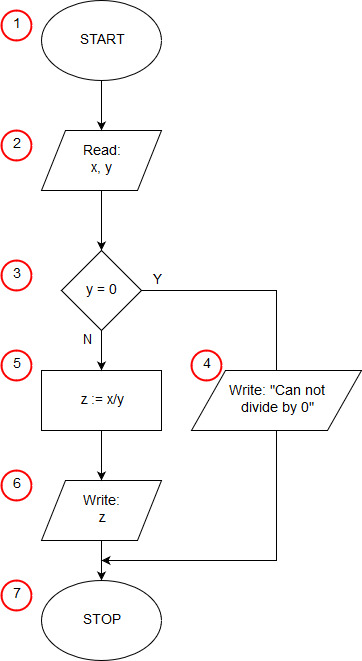# Algorithm of division in Pascal

<<algorithmprogram Division; var x, y, z: real; begin write('x = '); readln(x); write('y = '); readln(y); if y=0 then writeln('Can not divide by 0') else begin z:=x/y; writeln('z = ',z); end; readln; end.

The first line of the program can be treated as an ornament, but required by the syntax of the language pascal. Every program in the pascal must start with the keyword program preceding the name of the program, it adds nothing to the implementation of the algorithm. The second line is auxiliary. We know that our algorithm uses three variables that store real numbers. In the pass, all variables must be declared before the block of code starts. When declaring a variable, we must specify what type of data will be stored in it.

The third line containing the key word begin corresponds to the starting block (1) of our algorithm.

Lines 4 through 7 perform an input/output operation (2) in which the input data is taken - a dividend and a divisor.

The conditional statement from line 8 is the selection block (3) from our algorithm. The instruction checks whether y is equal to 0. When the condition is met, the instruction followed by the keyword then is stored in line 9 (in the algorithm of the input output block (4)). When the condition is not met, the statement after the else keyword is executed, in our case it is a compound statement, enclosed in beginners logical brackets between lines 10 and 13. First, in line 11 we calculate the quotient x by y (operation block (5)), then in line 12 we print the result obtained (input-output block (6)).

The readln statement on line 14 has nothing to do with the execution of the splitting algorithm. It serves to stop the program until you press the enter key, which prevents the program window from closing immediately.

Instructions end. from line 15, the program ends (end block of the algorithm (7)).